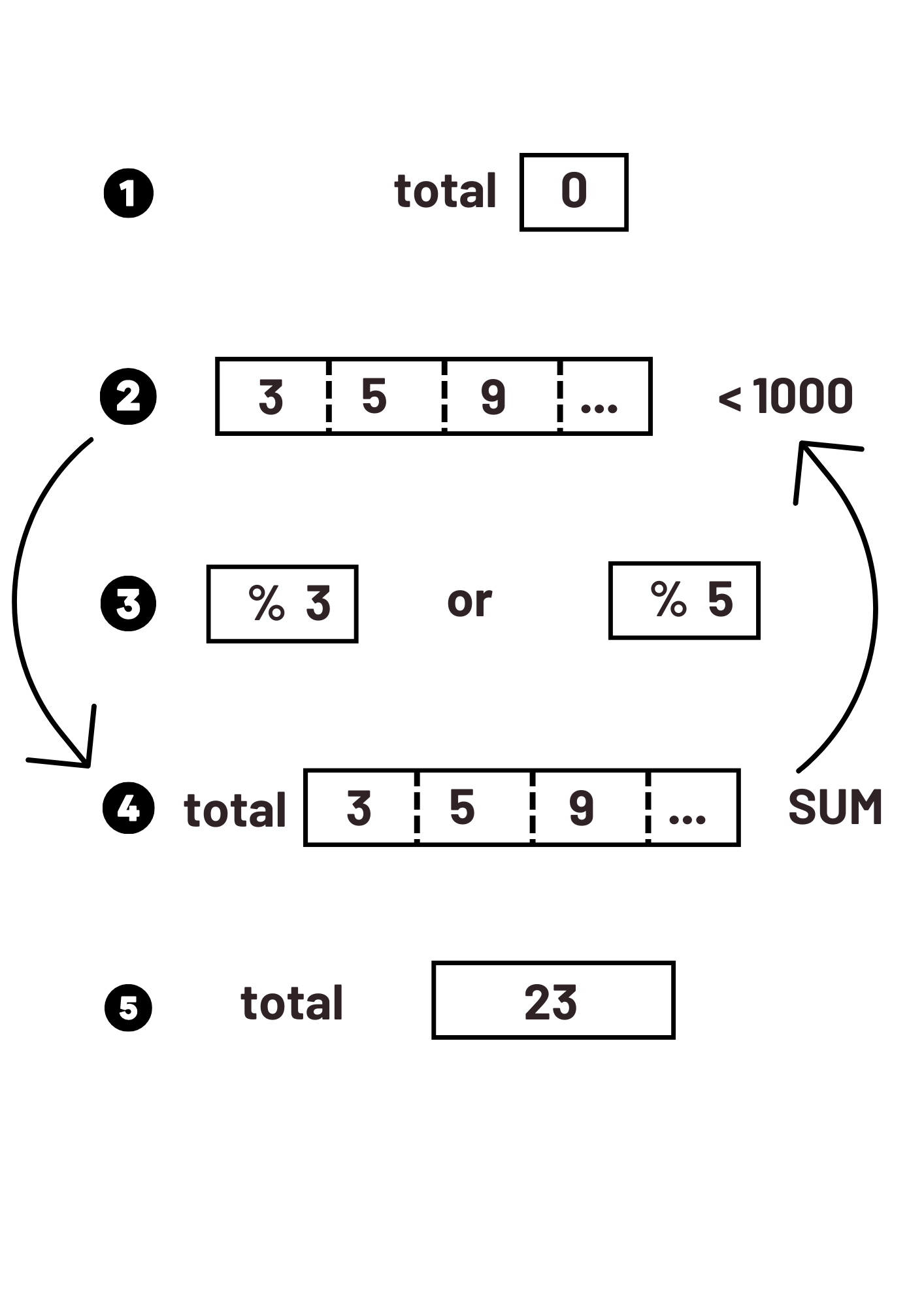# Multiples of 3 or 5

#### Statement

If we list all the natural numbers below 10 that are multiples of 3 or 5, we get 3, 5, 6 and 9. The sum of these multiples is 23. Find the sum of all the multiples of 3 or 5 below 1000.

#### Procedure

1. Initialize the total to zero

2. List the natural numbers below 1000

3. Use modulo to find the multiple of 3 or 5

4. Increment the counter to sum these multiples

5. Display the result

#### SchemaIn multiples, we find multiplication. So we will multiply 3 and 5 by all the integers up to 1000 number. It is enough to multiply this number by 1, 2, 3, 4, 5, etc.

Example: the multiples of 3 are: 3x1, 3x2, 3x3, 3x4, etc., which gives 3, 6, 9, 12, 15, 18, 21, etc.

The list of multiples of a number is infinite (we can't list them all!).

For 5, it is easy because it always ends with 5 or 0.

When we talk about multiplication, we also talk about division (9/3=0) and the remainder. The simple approach for this problem is to count on the remainder because if we get zero, the number is indeed multiple. For this, we will use the modulo. It's a perfect operator for that.

Finally, we need to recover the sum. We can therefore set up a counter system that we initialize at zero and then increment as we go along in the loop.

We get 233168.